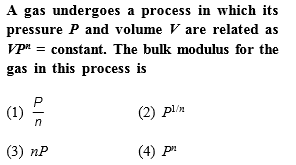[Only for Dropper and XII batch]

Complete Question Bank + Test Series
Complete Question Bank

Difficulty Level: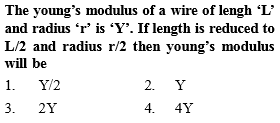Complete Question Bank + Test Series
Complete Question Bank

Difficulty Level: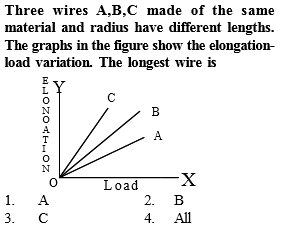Complete Question Bank + Test Series
Complete Question Bank

Difficulty Level: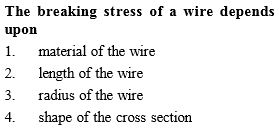Complete Question Bank + Test Series
Complete Question Bank

Difficulty Level: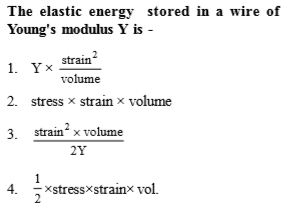Complete Question Bank + Test Series
Complete Question Bank

Difficulty Level: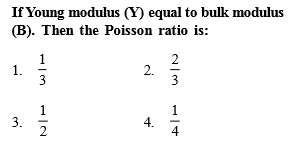Complete Question Bank + Test Series
Complete Question Bank

Difficulty Level:

The bulk modulus of a spherical object is B. If it is subjected to uniform pressure p, the fractional decrease in radius is

(a)$\frac{P}{B}$

(b) $\frac{B}{3p}$

(c) $\frac{3p}{B}$

(d)$\frac{p}{3B}$

Complete Question Bank + Test Series
Complete Question Bank

Difficulty Level:

The Young's modulus of steel is twice that of brass. Two wires of same length and of same area of cross-section, one of steel and another of brass are suspended from the same roof. If we want the lower ends of the wires to be at the same level, then the weight added to the steel and brass wires must be in the ratio of

(a)1:2

(b)2:1

(c)4:1

(d)1:1

Complete Question Bank + Test Series
Complete Question Bank

Difficulty Level:

Copper of fixed volume V is drawn into wire of length l. When this wire is subjected to a constant force F, the extension produced in the wire is  Δl. Which of the following graphs is a straight line?

(a)Δl versues 1/l

(b)Δl versus l2

(c)Δl versus 1/l2

(d) Δl versus l

Complete Question Bank + Test Series
Complete Question Bank

Difficulty Level:

The following four wires are made of the same material. Which of then will have the largest extension when the same tension is applied?

(a) Length=50cm, diameter=0.5mm

(b) Length=100cm, diameter=1mm

(c) Length=200cm, diameter=2mm

(d) Length=300cm, diameter=3mm